# Circles and Stars

Sophie is playing a game called Circles and Stars. Sophie rolls one number cube and gets the number six. Sophie draws six circles on her paper. Sophie rolls the number cube again and gets the number three. Sophie draws three stars in each of the six circles. Sophie writes 6 x 3 on her paper. Sophie plays the game again. Sophie rolls one number cube and gets the number three. Sophie draws three circles on her paper. Sophie rolls the number cube again and gets the number six. Sophie draws six stars in each of the three circles. Sophie writes 3 x 6 on her paper. Sophie says she got the same total number of stars both times! Is Sophie correct? Show all your mathematical thinking.

Assessment

## Plan

#### Formal Mathematical Language and Symbolic Notation

A student may independently select a printed number line, number chart, ten frames, graph paper, etc. as they work on a task.

### Suggested materials

Engagement Image:

Teachers may project the images below to launch this task for their students, define nouns, promote discussion, access prior knowledge, and inspire engagement and problem solving.

## Solutions tabs

### Expert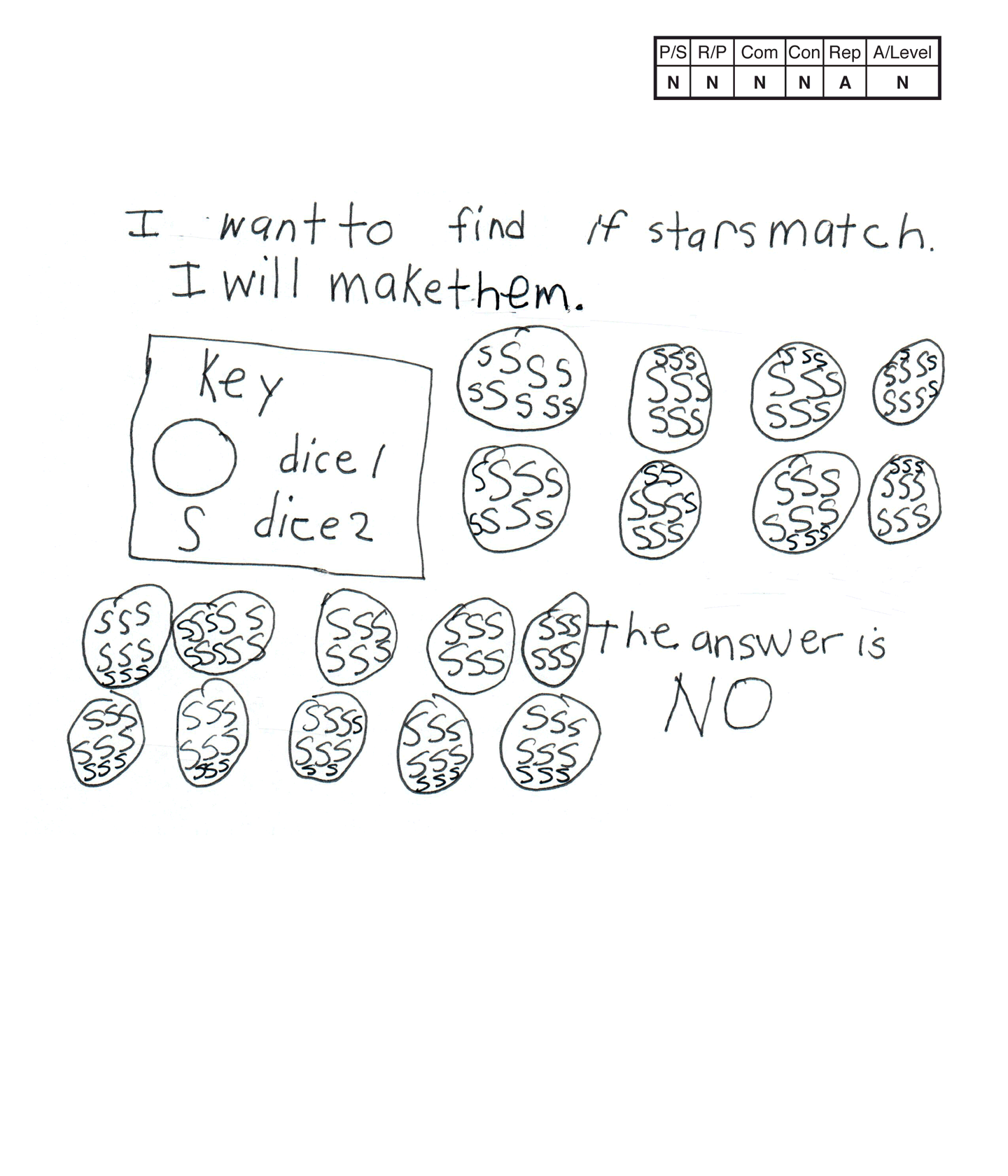This student does not meet the standard.

### Scoring Rationale

#### Novice

The student's strategy of diagramming 18 circles with nine S's (stars?) in each circle would not work to solve the task. The student's answer, "NO," is not correct.

#### Novice

The student does not show correct reasoning of the underlying concepts of the task. The student is not able to place three stars in each of six circles or six stars in each of three circles. It appears that the student may have added six and three for a total of nine stars and multiplied six and three for a product of 18 circles to form the structure of their diagram.

#### Novice

The student does not earn credit for the term key because it is used incorrectly in the student's diagram.

#### Novice

The student does not make a mathematically relevant observation about their solution.

#### Apprentice

The student attempts to make a diagram but it is not accurate. The student diagrams 18 circles with nine S's (stars?) in each circle. The student's diagram does not record or communicate correct reasoning.

#### Novice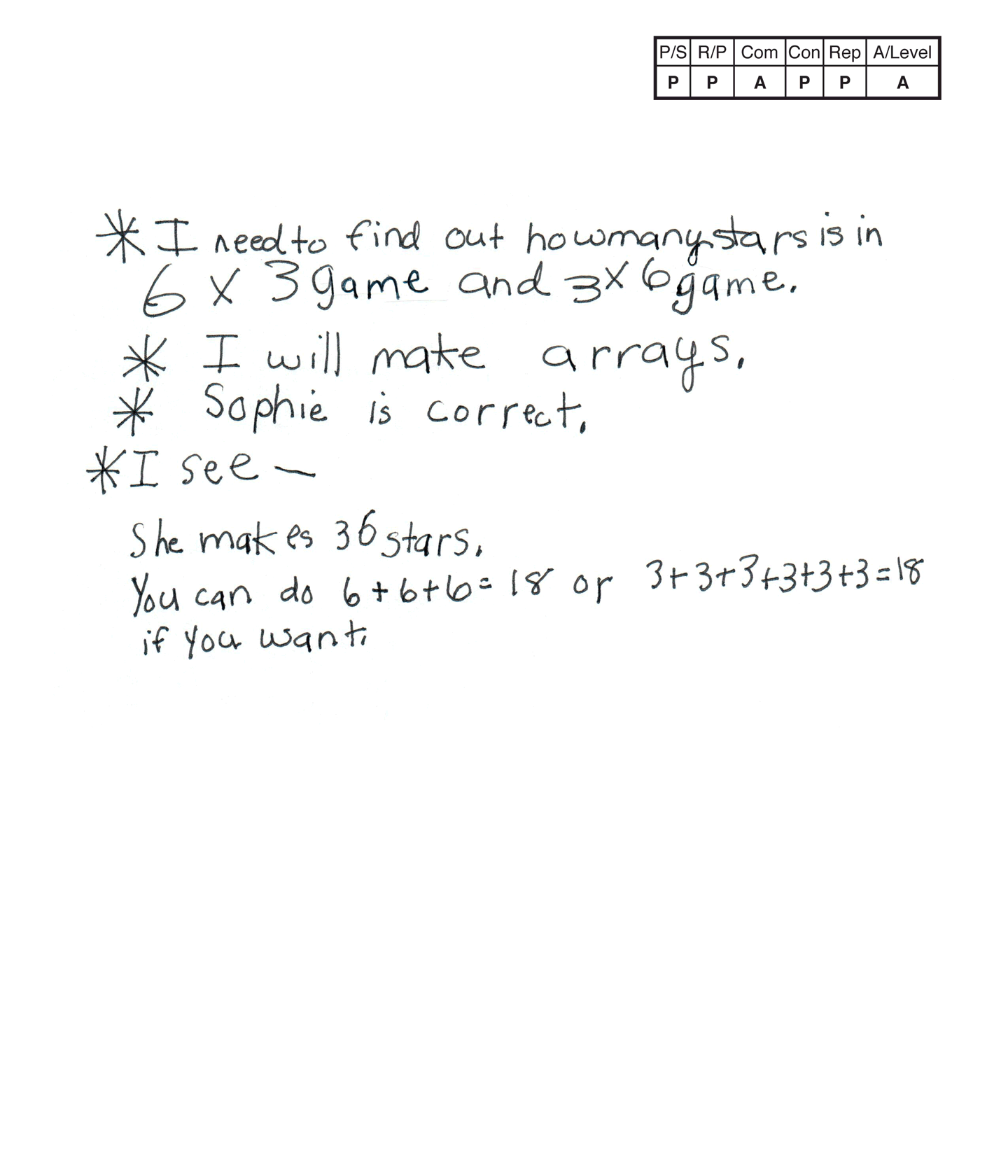,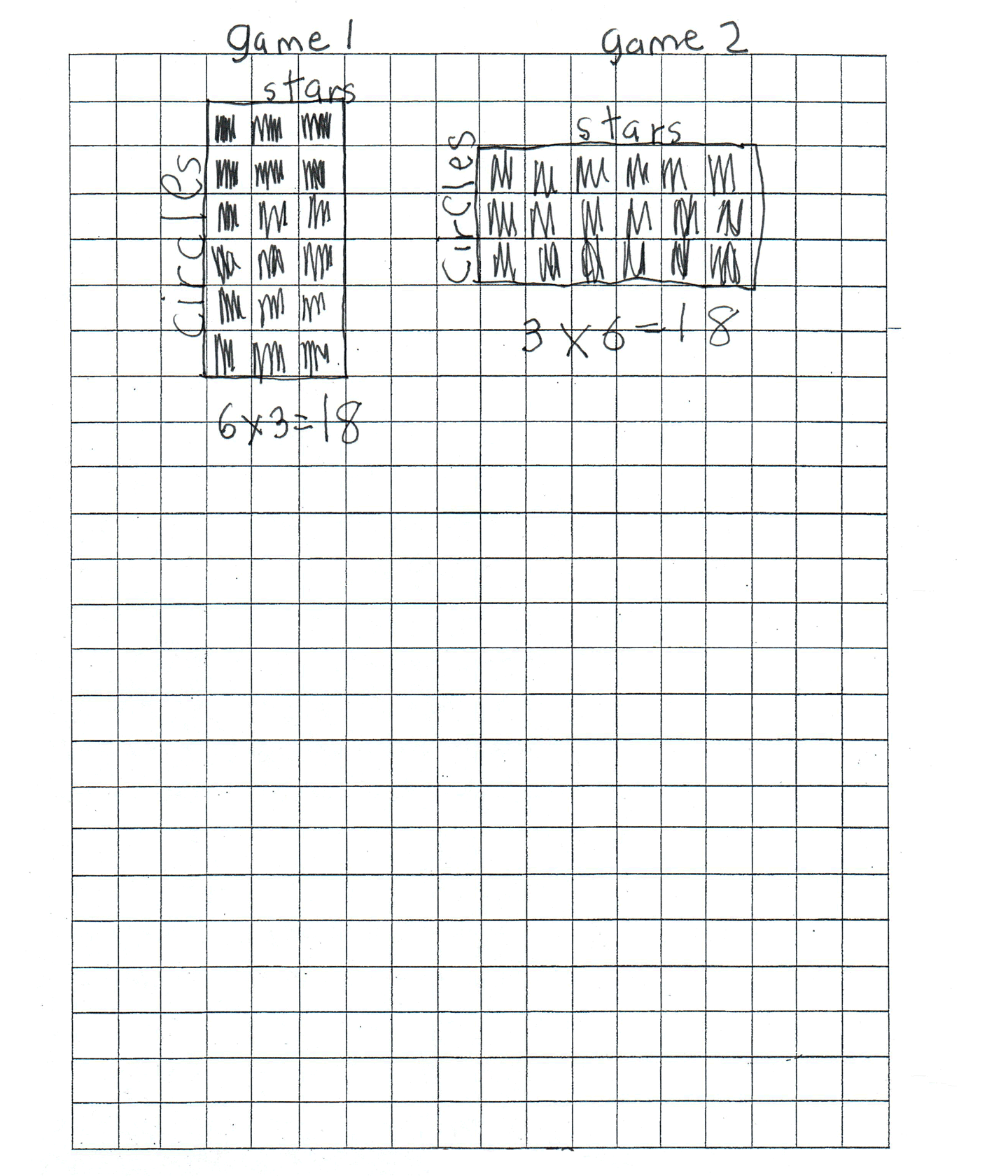This student does not meet the standard.

### Scoring Rationale

#### Practitioner

The student's strategy of making two arrays to determine that 6 x 3 and 3 x 6 have the same product works to solve the task. The student's answer, "Sophie is correct," is correct.

#### Practitioner

The student shows correct reasoning of the underlying concepts of the task. The student uses arrays to confirm that 18 stars is the product for the first and second game number rolls.

#### Apprentice

The student correctly uses the mathematical term array.

#### Practitioner

The student makes mathematically relevant observations about their solution by stating, "She makes 36 stars," and, "You can do 6 + 6 + 6 = 18 or 3 + 3 + 3 + 3 + 3 + 3 = 18 if you want."

#### Practitioner

The student's arrays are appropriate to the task and accurate. The circles and stars are labeled and the arrays are correctly constructed.

#### Apprentice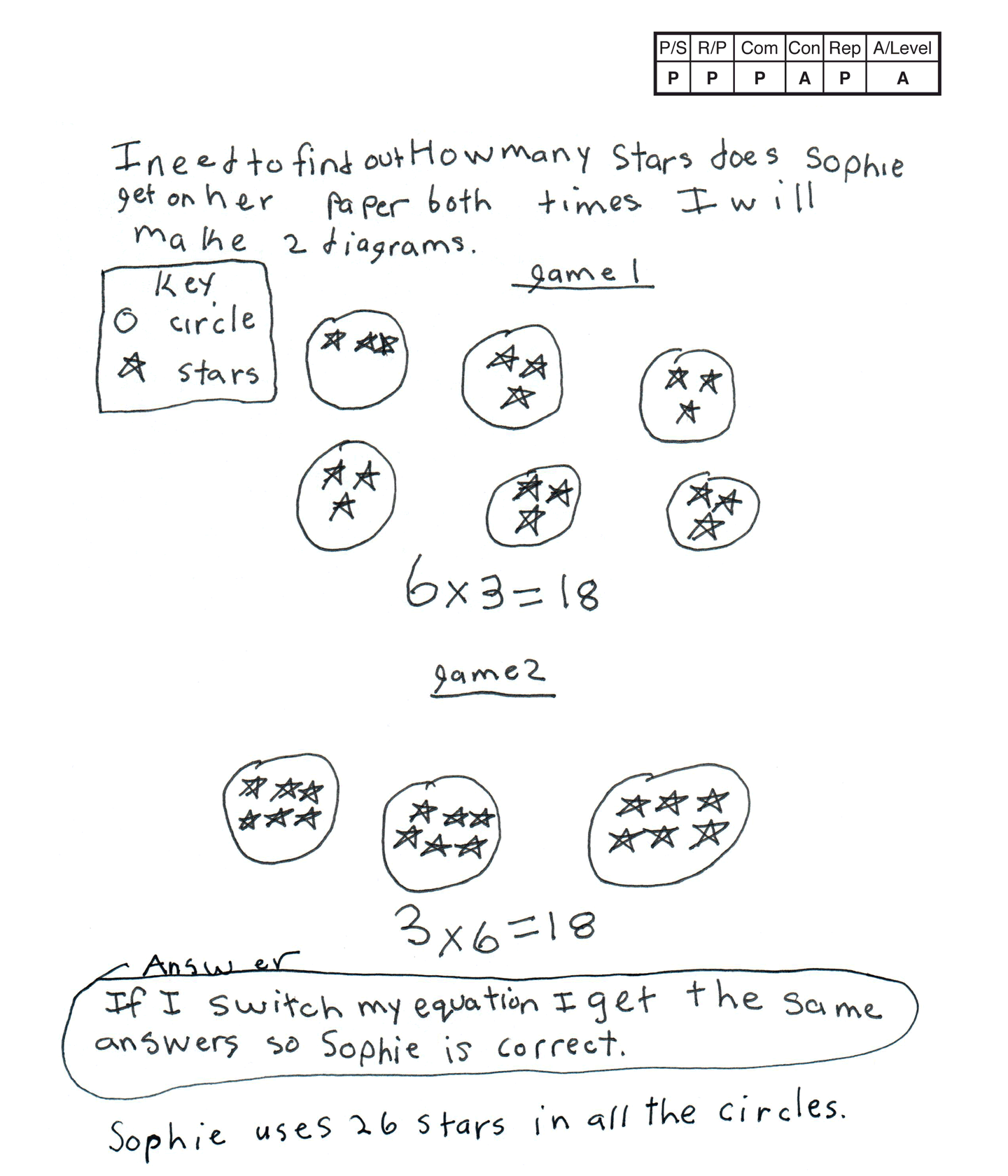This student does not meet the standard.

### Scoring Rationale

#### Practitioner

The student's strategy of making two diagrams to determine that 6 x 3 and 3 x 6 have the same product works to solve the task. The student's answer, "If I switch my equation I get the same answers so Sophie is correct," is correct.

#### Practitioner

The student shows correct reasoning of the underlying concepts of the task. The student uses diagrams to confirm that 18 stars is the product for the first and second game number rolls.

#### Practitioner

The student correctly uses the mathematical terms diagrams, key, circle, equation.

#### Apprentice

The student attempts to make a mathematically relevant observation about their solution by stating, "Sophie uses 26 stars in all the circles." The student may have regrouped incorrectly arriving at an incorrect number in the tens place.

#### Practitioner

The student's diagrams are appropriate to the task and accurate. The circles and stars are labeled by a key and each circle contains the correct number of stars.

#### Apprentice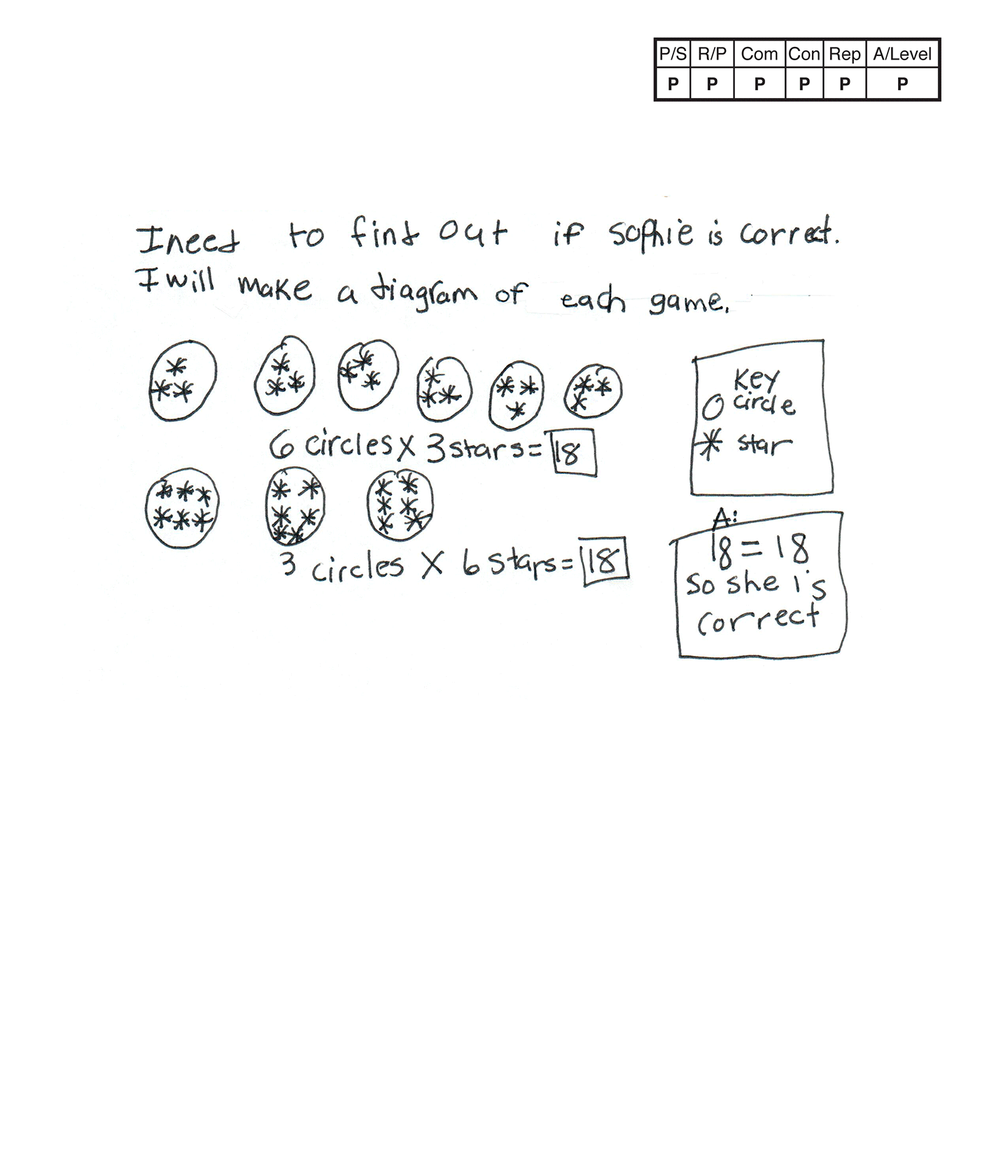,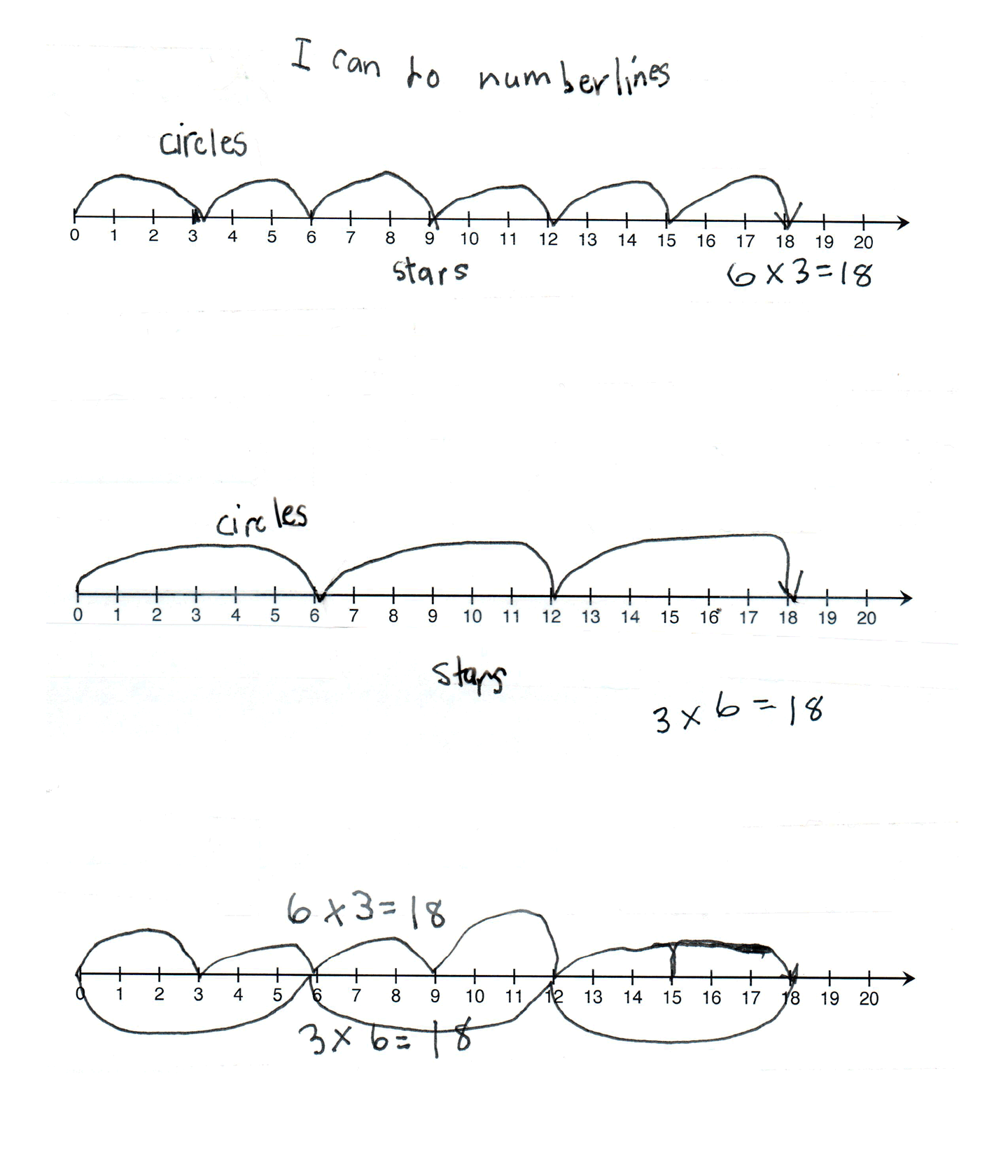This student meets the standard.

### Scoring Rationale

#### Practitioner

The student's strategy of making diagrams to determine that 6 x 3 and 3 x 6 have the same product works to solve the task. The student's answer, "18 = 18 so she is correct," is correct.

#### Practitioner

The student shows correct reasoning of the underlying concepts of the task. The student uses diagrams to confirm that 18 stars is the product for the first and second game number rolls.

#### Practitioner

The student correctly uses the mathematical terms diagrams, key, circle, number line.

#### Practitioner

The student uses a different strategy to compare 6 x 3 and 3 x 6 by making three number lines. The student does not earn Expert credit for verification because the student does not link the two strategies/representations to confirm that Sophie was correct.

#### Practitioner

The student's diagrams are appropriate to the task and accurate. The circles and stars are labeled in a key and each circle contains the correct number of stars. The student's first two number lines are also appropriate to the task and accurate. The stars and circles are labeled and the jumps are correctly indicated. The student's third number line lacks labels. The 3 x 6 "jumps" should be above the number line to be accurate. The student is using the number line as a diagram which is a creative strategy but it needs labels.

#### Practitioner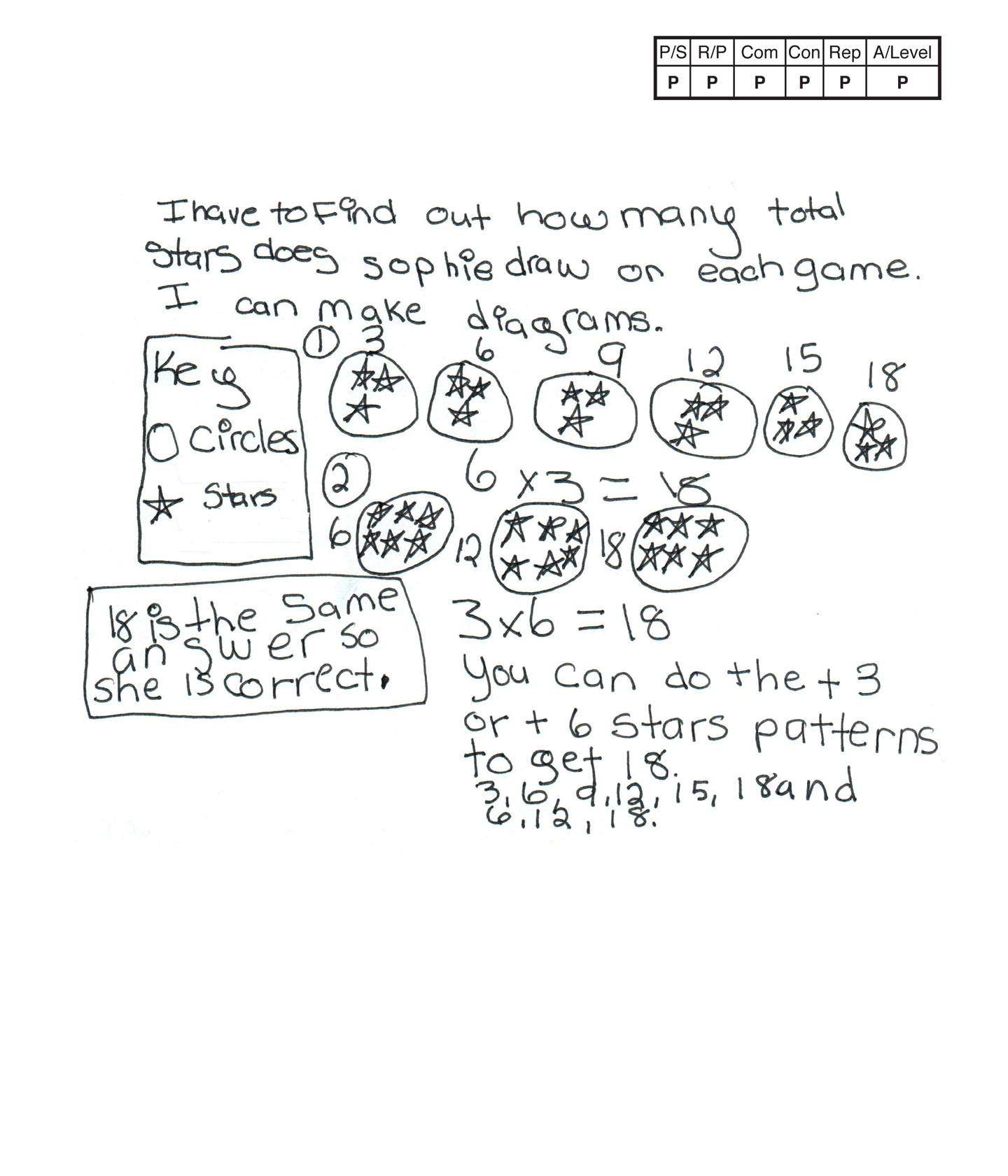This student meets the standard.

### Scoring Rationale

#### Practitioner

The student's strategy of making diagrams to determine that 6 x 3 and 3 x 6 have the same product works to solve the task. The student's answer, "18 is the same answer so she is correct," is correct.

#### Practitioner

The student shows correct reasoning of the underlying concepts of the task. The student uses diagrams to confirm that 18 stars is the product for the first and second game number rolls.

#### Practitioner

The student correctly uses the mathematical term total from the task. The student also correctly uses the terms diagrams, key, circle, patterns.

#### Practitioner

The student makes the mathematically relevant observation, "You can do the + 3 or + 6 stars patterns to get 18. 3, 6, 9, 12, 15, 18 and 6, 12, 18."

#### Practitioner

The student's diagrams are appropriate to the task and accurate. The circles and stars are labeled by a key and each circle contains the correct number of stars.

#### Practitioner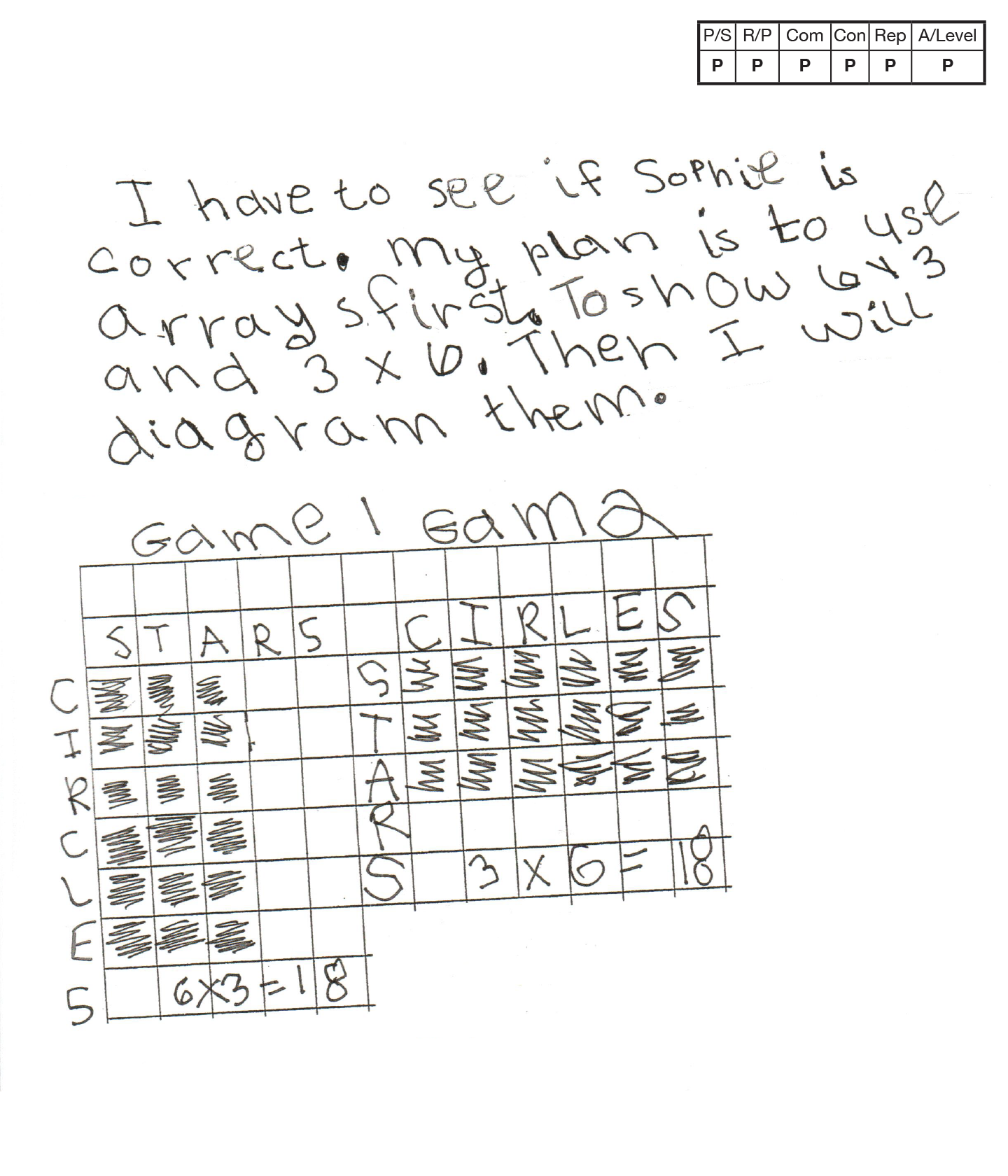,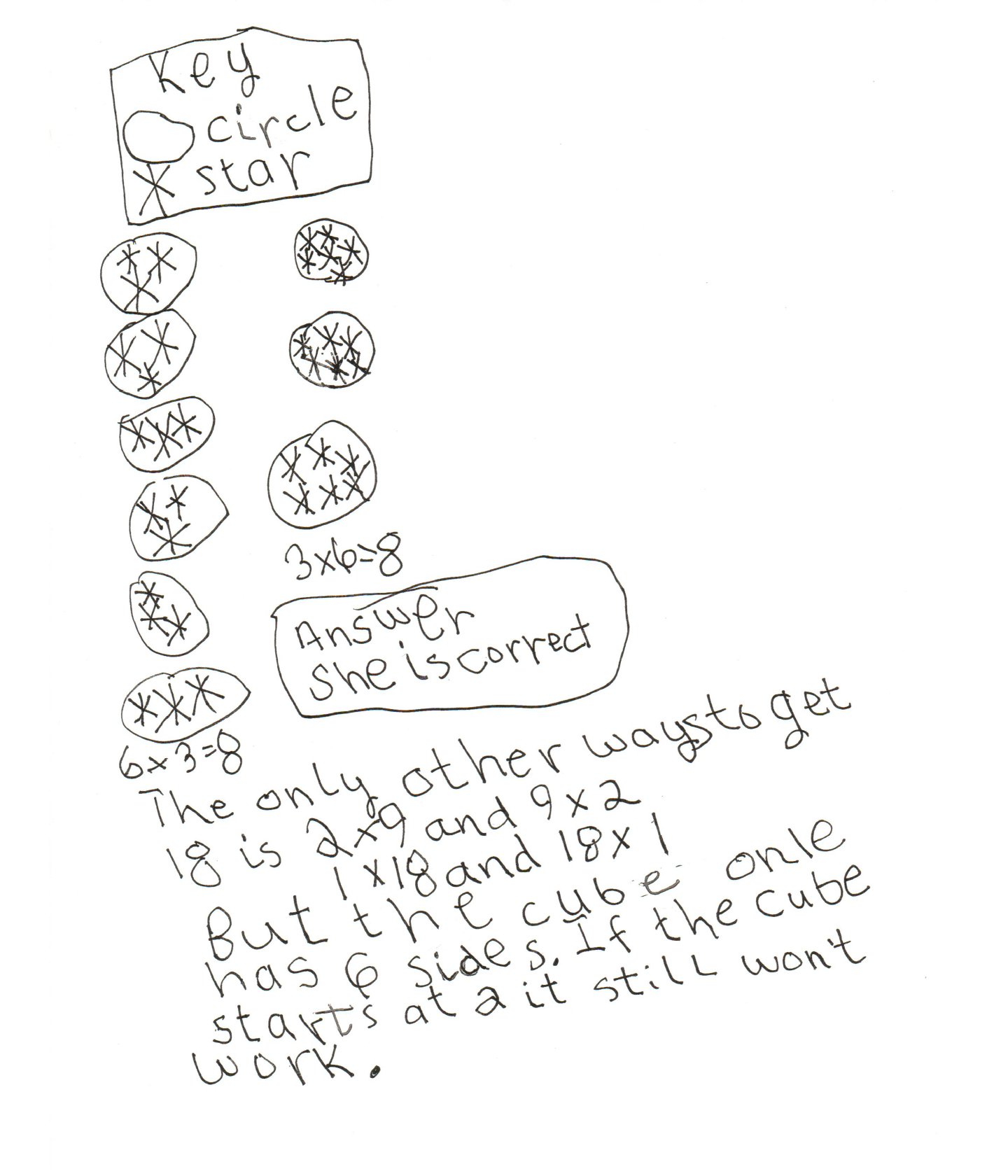This student meets the standard.

### Scoring Rationale

#### Practitioner

The student's strategies of using arrays and a diagram to determine that 6 x 3 and 3 x 6 have the same product works to solve the task. The student's answer, "She is correct," is correct.

#### Practitioner

The student shows correct reasoning of the underlying concepts of the task. The student uses arrays and diagrams to confirm that 18 stars is the product for the first and second game number rolls.

#### Practitioner

The student correctly uses the mathematical terms array, diagram, key, cube.

#### Practitioner

The student makes mathematically relevant observations, "The only other ways to get 18 is 2 x 9 and 9 x 2, 1 x 18 and 18 x 1. But the cube onle has 6 sides. If the cube starts at 2 is still won't work."

#### Practitioner

The student's arrays are appropriate to the task and accurate. Labels provide the necessary information. The circles and stars are defined by a key and each circle contains the correct number of stars.

#### Practitioner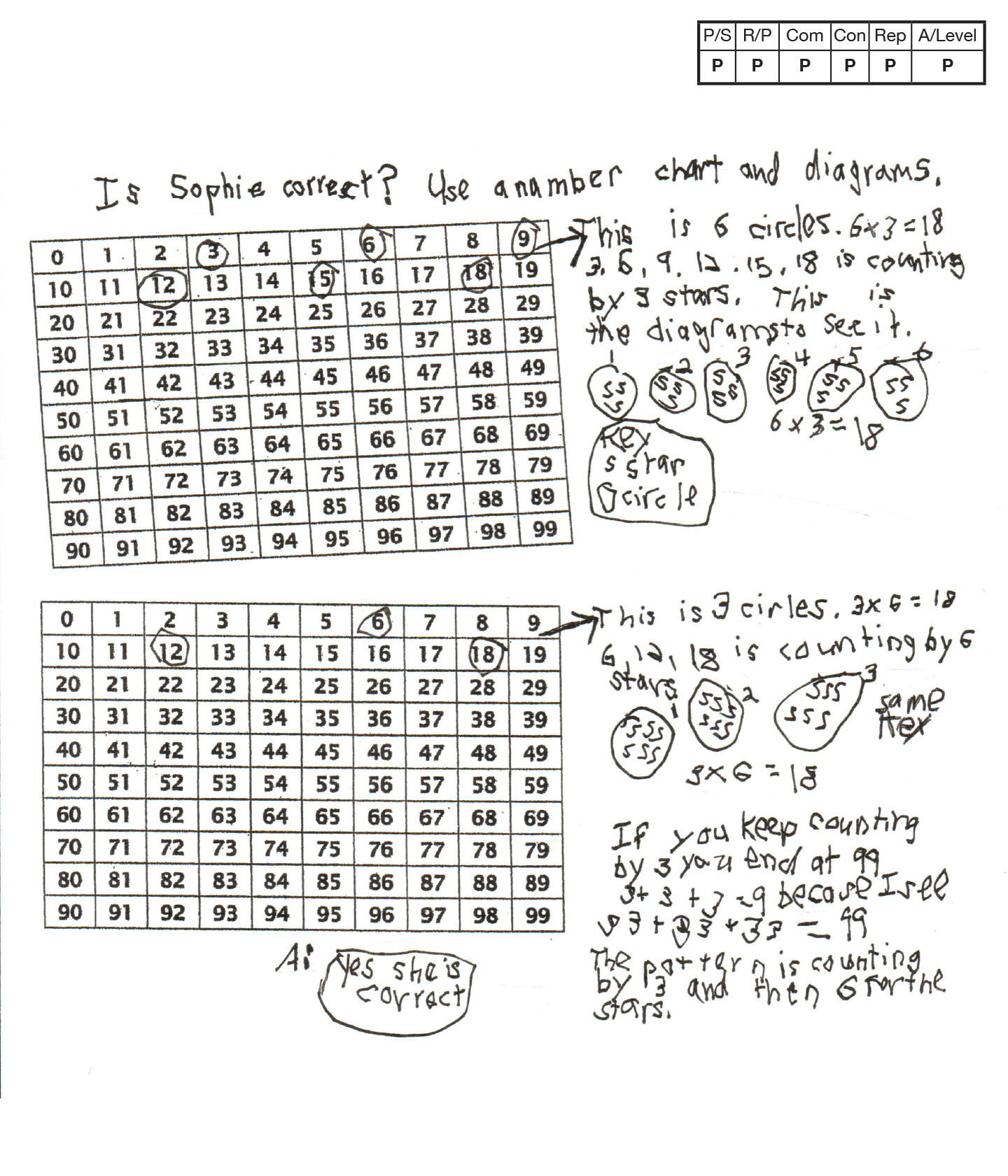This student meets the standard.

### Scoring Rationale

#### Practitioner

The student's strategies of using number charts and diagrams to determine that 6 x 3 and 3 x 6 have the same product works to solve the task. The student's answer, "Yes she is correct," is correct.

#### Practitioner

The student shows correct reasoning of the underlying concepts of the task. The student uses number charts and diagrams to confirm that 18 stars is the product for the first and second game number rolls.

#### Practitioner

The student correctly uses the mathematical terms number chart, circles, diagrams, key, pattern.

#### Practitioner

The student makes the mathematically relevant observations, "If you keep counting by 3 you end at 99. 3 + 3 + 3 = 9 because I see 33 + 33 + 33 = 99" and, "The pattern is counting by 3 and then 6 for the stars."

#### Practitioner

The student's use of number charts to find the muliples of three and six is appropriate to the task and accurately labeled with arrows and a written explanation. The student's diagrams of circles and stars are defined by a key and each circle contains the correct number of stars.

#### Practitioner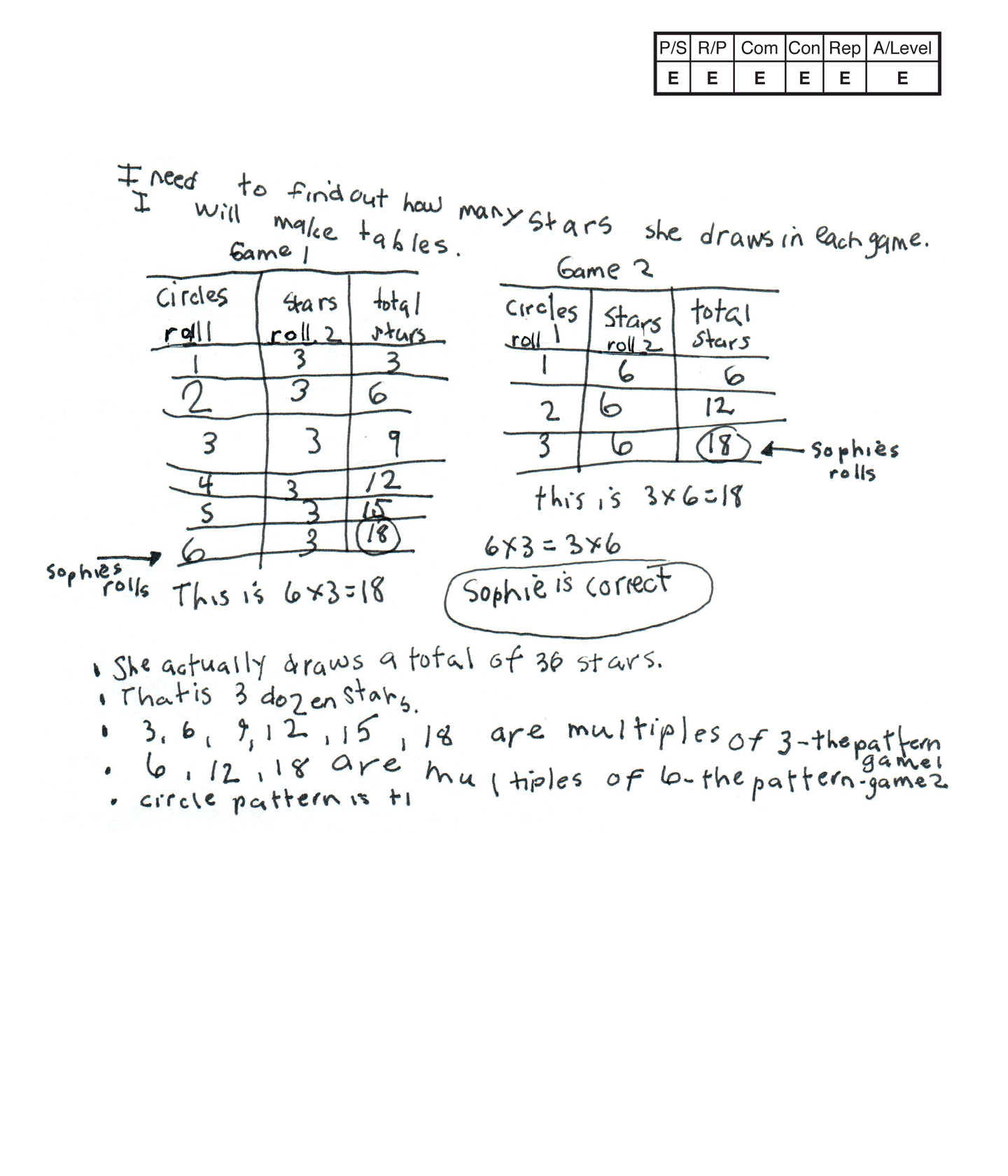,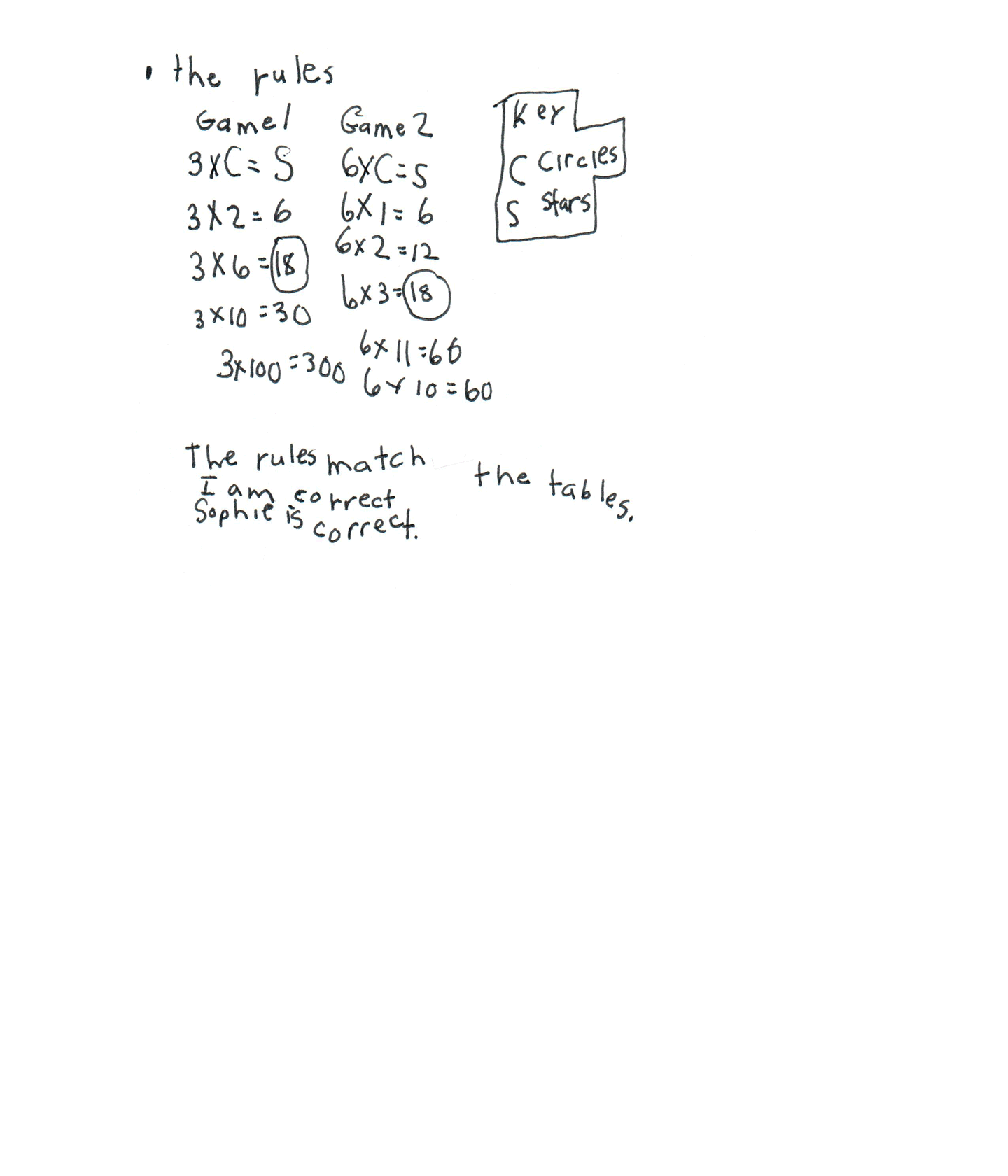This student exceeds the standard.

### Scoring Rationale

#### Expert

The student's strategy of making tables to determine that 6 x 3 and 3 x 6 have the same product works to solve the task. The student's answer, "Sophie is correct," is correct. The student verifies their answer by generalizing and proving two rules.

#### Expert

The student shows correct reasoning of the underlying concepts of the task. The student uses tables to confirm that 18 stars is the product for the first and second game number rolls. The student justifies that their answer is correct by applying two rules, 3 x C = S and 6 x C = S.

#### Expert

The student correctly uses the mathematical term total from the task. The student also correctly uses the terms tables, dozen, multiples, pattern, rules, key, circles. The student uses the symbolic notation 3 x C = S, 6 x C = S.

#### Expert

The student makes the mathematically relevant Practitioner observations, "She actually draws a total of 36 stars," "That is 3 dozen stars," "3, 6, 9, 12, 15, 18 are multiples of 3-the pattern game 1," "6, 12, 18 are multiples of 6-the pattern game 2," and, "circle pattern is +1." The student makes the Expert connection by verifying that their answer is correct. The student generalizes the rules using defined variables, 3 x C = S and 6 x C = S. The student uses the rules to solve for two, six, 10, and 100 circles with three per circle and one, two, three, 11, and 10 circles with six stars per circle. The student states, "The rules match the tables. I am correct, Sophie is correct."

#### Expert

The student's tables are appropriate to the task and accurate. All labels are provided and the data is correct. The student uses their tables to generalize two rules to support the data on the tables and to verify that their answer is correct. The student defines the variables in their key.